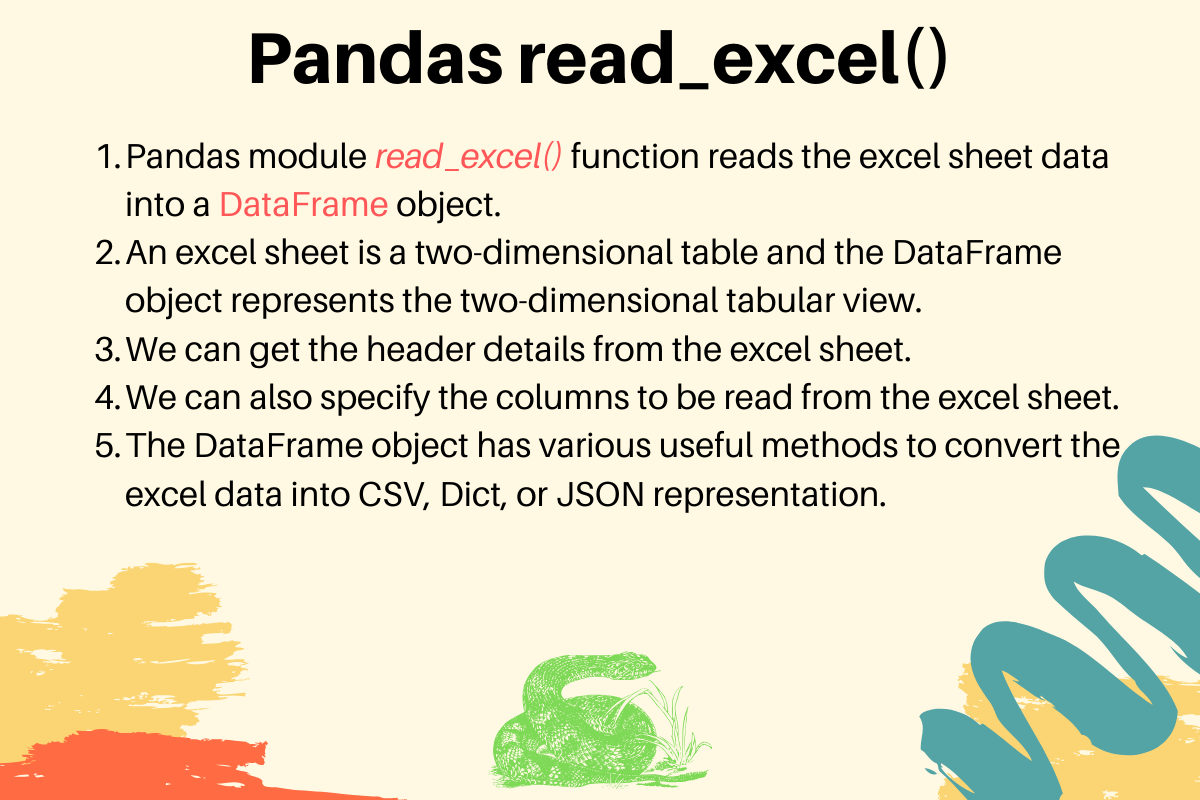#### // Tutorial //

Published on August 3, 2022By Pankaj
Developer and author at DigitalOcean.While we believe that this content benefits our community, we have not yet thoroughly reviewed it. If you have any suggestions for improvements, please let us know by clicking the “report an issue“ button at the bottom of the tutorial.

We can use the pandas module read_excel() function to read the excel file data into a DataFrame object. If you look at an excel sheet, it’s a two-dimensional table. The DataFrame object also represents a two-dimensional tabular data structure.

Let’s say we have an excel file with two sheets - Employees and Cars. The top row contains the header of the table.

Here is the example to read the “Employees” sheet data and printing it.

``````import pandas

# print whole sheet data
print(excel_data_df)
``````

Output:

``````   EmpID    EmpName EmpRole
0      1     Pankaj     CEO
1      2  David Lee  Editor
2      3   Lisa Ray  Author
``````
• The first parameter is the name of the excel file.
• The sheet_name parameter defines the sheet to be read from the excel file.
• When we print the DataFrame object, the output is a two-dimensional table. It looks similar to an excel sheet records.

## 2. List of Columns Headers of the Excel Sheet

We can get the list of column headers using the `columns` property of the dataframe object.

``````print(excel_data_df.columns.ravel())
``````

Output:

``````['EmpID' 'EmpName' 'EmpRole']
``````

## 3. Printing a Column Data

We can get the column data and convert it into a list of values.

``````print(excel_data_df['EmpName'].tolist())
``````

Output:

``````['Pankaj', 'David Lee', 'Lisa Ray']
``````

## 4. Pandas read_excel() usecols example

We can specify the column names to be read from the excel file. It’s useful when you are interested in only a few of the columns of the excel sheet.

``````import pandas

excel_data_df = pandas.read_excel('records.xlsx', sheet_name='Cars', usecols=['Car Name', 'Car Price'])
print(excel_data_df)
``````

Output:

``````         Car Name      Car Price
0      Honda City     20,000 USD
1  Bugatti Chiron  3 Million USD
2     Ferrari 458   2,30,000 USD
``````

If the excel sheet doesn’t have any header row, pass the header parameter value as None.

``````excel_data_df = pandas.read_excel('records.xlsx', sheet_name='Numbers', header=None)
``````

If you pass the header value as an integer, let’s say 3. Then the third row will be treated as the header row and the values will be read from the next row onwards. Any data before the header row will be discarded.

## 6. Excel Sheet to Dict, CSV and JSON

The DataFrame object has various utility methods to convert the tabular data into Dict, CSV, or JSON format.

``````excel_data_df = pandas.read_excel('records.xlsx', sheet_name='Cars', usecols=['Car Name', 'Car Price'])

print('Excel Sheet to Dict:', excel_data_df.to_dict(orient='record'))
print('Excel Sheet to JSON:', excel_data_df.to_json(orient='records'))
print('Excel Sheet to CSV:\n', excel_data_df.to_csv(index=False))
``````

Output:

``````Excel Sheet to Dict: [{'Car Name': 'Honda City', 'Car Price': '20,000 USD'}, {'Car Name': 'Bugatti Chiron', 'Car Price': '3 Million USD'}, {'Car Name': 'Ferrari 458', 'Car Price': '2,30,000 USD'}]
Excel Sheet to JSON: [{"Car Name":"Honda City","Car Price":"20,000 USD"},{"Car Name":"Bugatti Chiron","Car Price":"3 Million USD"},{"Car Name":"Ferrari 458","Car Price":"2,30,000 USD"}]
Excel Sheet to CSV:
Car Name,Car Price
Honda City,"20,000 USD"
Bugatti Chiron,3 Million USD
Ferrari 458,"2,30,000 USD"
``````

## 7. ReferencesPankaj

author

Developer and author at DigitalOcean.

#### Still looking for an answer?

great work… thanks a lot for posting

- Rengifo

how can we remove a specific row? I have a ‘Total’ row at the end of my Excel file that I would want to remove. Thanks for the helpful info in this article!

- Josh Rodriguez

Thanks pankaj… It saved my data. I wanted to read from a specific folder so I tweaked it for the location. pd.read_excel(‘C:/Source/Datafile.xlsx’, sheet_name=‘sheet_name’)

- Rajjan

Thanks, useful post. excel_data_df = pandas.read_excel(‘records.xlsx’, sheet_name=‘Cars’, usecols=[‘Car Name’, ‘Car Price’]) results in an empty dataframe for me. Do we need to do some setup?

- Rahul Misra

I must say this is ‘awesome’

- Prabhat Kumar

- Havi

Thank you, it was very helpful

- mila

- byli

Thanks it helped me

- firozsahib

What is we have an xlsb file instead of xlsx?

- Shilpa Bindra